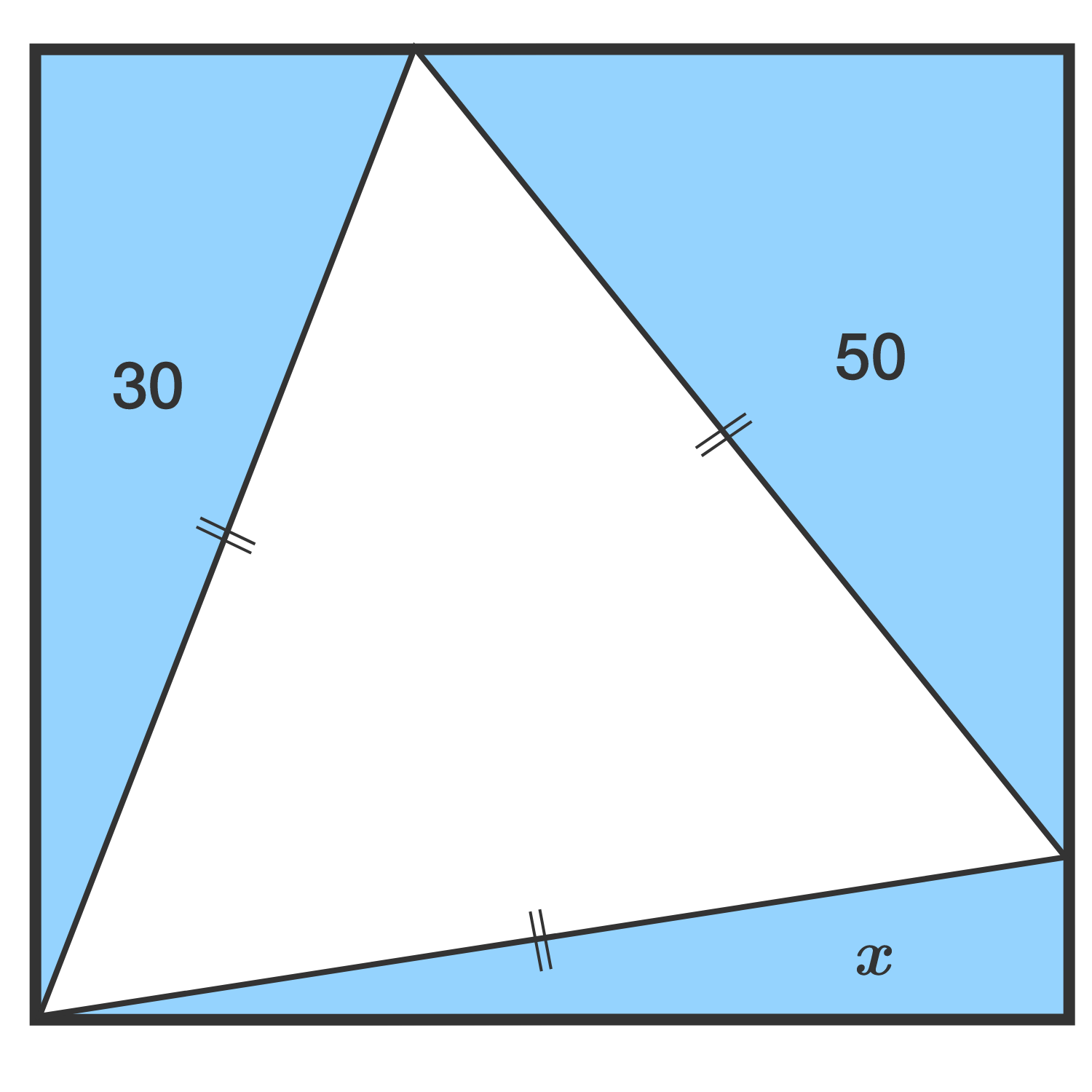# An Inscribed Equilateral Triangle

Geometry Level 2

As shown, a rectangle (not a square) is partitioned into four triangles: 1 equilateral and 3 right triangles. The areas of two of the right triangles are 30 and 50. Find the area $x$ of the remaining right triangle.Bonus: Find the general relationship among the areas of the 3 right triangles.

×

Problem Loading...

Note Loading...

Set Loading...